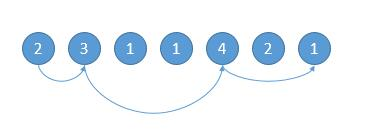# 题目描述（困难难度）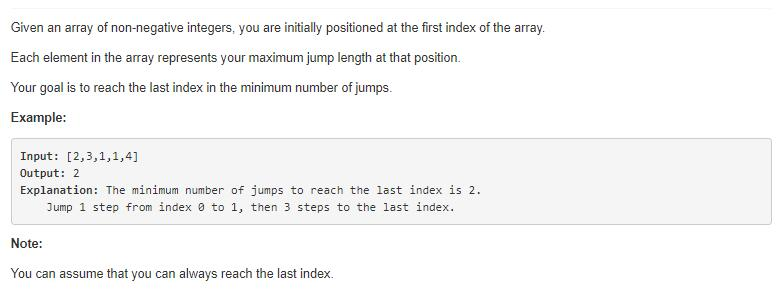# 解法一 顺藤摸瓜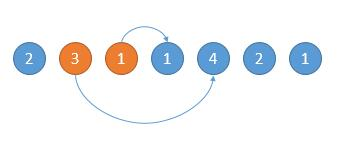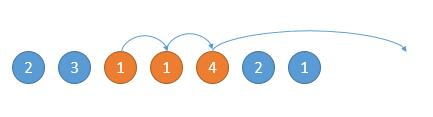public int jump(int[] nums) {
int end = 0;
int maxPosition = 0;
int steps = 0;
for(int i = 0; i < nums.length - 1; i++){
//找能跳的最远的
maxPosition = Math.max(maxPosition, nums[i] + i);
if( i == end){ //遇到边界，就更新边界，并且步数加一
end = maxPosition;
steps++;
}
}
return steps;
}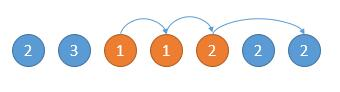# 解法二 顺瓜摸藤

public int jump(int[] nums) {
int position = nums.length - 1; //要找的位置
int steps = 0;
while (position != 0) { //是否到了第 0 个位置
for (int i = 0; i < position; i++) {
if (nums[i] >= position - i) {
position = i; //更新要找的位置
steps++;
break;
}
}
}
return steps;
}